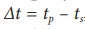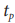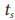Erasmus+: Key action 2:Strategic Partnerships in School Education# INTRODUCTION

Measuring of time is a topic that occurs in primary school curriculum, albeit in its most basic form, already in the introductory years. The astronomical knowledge on which the time measurement is based is then divided according to the development of intellectual abilities of children with age in the curriculum in subsequent years, practically throughout primary school, but also subsequently in other levels of education.

# Apparent Solar Time and Mean Solar Time

Astronomical phenomena allow people to break down the continuous flow of time into different lengths, but usually longer periods of time. Various astronomical bodies can be used for this division, but the most significant from the point of view of observers is the Sun and the Moon. Over time, connections between basic time units derived from the movements of astronomical bodies across the sky were sought, and the first calendars were created. At the same time, the values of the basic time units were improved, and with a deeper understanding of astronomical phenomena and processes, other time units were created. The industry development has led to the invention of various timekeeping devices – clocks – and to the need to introduce more accurate time measurement, especially in the area of shorter time intervals.

## Sun and Time Measurement

As mentioned above, the most striking body in the sky is the Sun. The movement of the Sun across the sky divides the time continuum into a regular alternation of days and nights. However, this division of the continuum is rough, and so the need arose to divide the day into shorter sections. The individual sections can be given by the different position of the sun disk in the sky. However, this monitoring of time periods is not entirely optimal, as it depends on the observer's location (for example, the position of the Sun over a lone tree may occur at different times of the day for the observer in different places). Watching the shadow of a rod driven into the ground proved to be more appropriate. This creates the first simple (from our point of view today, more precisely gnomonic) sundial.

The time, which is measured by the sundial, is derived from the movement of the solar disk across the sky (more precisely, the rotation of the Earth around the axis relative to the Sun) and is referred to as apparent solar time. The term apparent sun is defined as the centre of a real solar disk in the sky. The apparent solar time is determined by the hour angle of the Sun and is divided into solar days and sundials. One solar day is the time that elapses during one rotation of the Earth, so it is the time between two passages of the Sun by a certain meridian. It can also be defined as the time between two consecutive culminations of the apparent sun. Because the sun's movement across the sky is uneven, the length of a apparent solar day changes throughout the year. For normal use, it is not completely optimal, and therefore in everyday life we use time derived from the mean solar time instead, which flows evenly.

There are two reasons for the unevenness of an apparent solar day: on the one hand, the elliptical trajectory of the Earth around the Sun, and on the other hand, the inclination of the Earth's axis (this cause, although mentioned second, is more significant for the unevenness). The shortest apparent solar day occurs on September 16 and is 21 s shorter than the average apparent solar day, while the longest apparent solar day occurs on December 22 and is 30 s longer than the average.

While the difference of tens of seconds in the measurement of time was of no significance to humanity (and especially until this difference was detectable at the time), the practical use of an apparent solar day was unproblematic. However, this ended with the development of accurate timekeeping. Then there was the need to use a uniform flowing time, but in such a way that, in principle, even with this uniform time, the length of the solar time did not change. This created the mean solar time (averaged apparent solar time), which contains the same number of seconds per year as the apparent solar time, but flows evenly.

## Mean Solar Time

It can be defined exactly so that it is an hour angle called the second mean sun. The second mean sun is an imaginary point in the celestial sphere, which moves evenly along the ecliptic and meets the apparent sun at the spring point. In addition, there is the first mean sun, which also moves evenly across the celestial sphere, but meets the apparent sun in perihelion and aphelion. Obviously, the mean solar time defined in this way is not entirely practical for determining from the motion of the sun, because the second mean sun is only an imaginary point. Therefore, in practice, the mean solar time is determined from the sidereal time, which will be discussed later. Even the mean solar time is not perfectly constant, especially with long-term time measurements exceeding several centuries (or with regard to changes caused by earthquakes, meteorite impacts on the earth's surface, or other similar phenomena). Because we can currently measure time with unprecedented accuracy, we are able to identify even small deviations. On average, the mean solar day is currently about 86,400.002 seconds defined in the SI system.(Source: https://en.wikipedia.org/wiki/File:Equation_of_time.svg)

## Time Equation

There are regular changes between apparent and mean solar time during the year, which are described by a physical quantity called the time equation. This is equal to the difference between the two times:, whereis the apparent solar time andis the mean solar time. The dependence of the time equation from the day of the year is shown in the attached graph. As the differences between the apparent and mean solar days gradually accumulate, the largest value of the time equation is 16.4 minutes (the apparent solar time is 16.4 minutes behind the mean solar time) on November 3. The minimum value of -14.4 minutes (apparent solar time is 14.4 minutes ahead of mean solar time) is reached by the time equation on February 12.